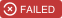## exponential-decay-histogram

A histogram which exponentially weights in favor of recent values

### 6 releases

 0.1.5 Jul 18, 2019 Mar 13, 2019 Nov 14, 2018 Sep 13, 2018 May 30, 2017

#207 in Data structures

Used in 6 crates (2 directly)

MIT/Apache

19KB
334 lines

# exponential-decay-histogramDocumentation

A histogram which exponentially weights in favor of recent values.

### Contribution

Unless you explicitly state otherwise, any contribution intentionally submitted for inclusion in the work by you, as defined in the Apache-2.0 license, shall be dual licensed as above, without any additional terms or conditions.

### `lib.rs`:

A histogram which exponentially weights in favor of recent values.

Histograms compute statistics about the distribution of values in a data set. This histogram exponentially favors recent values over older ones, making it suitable for use cases such as monitoring the state of long running processes.

The histogram does not store all values simultaneously, but rather a randomized subset. This allows us to put bounds on overall memory use regardless of the rate of events.

This implementation is based on the `ExponentiallyDecayingReservoir` class in the Java Metrics library, which is itself based on the forward decay model described in Cormode et al. 2009.

# Examples

``````use exponential_decay_histogram::ExponentialDecayHistogram;

# fn do_work() -> i64 { 0 }
let mut histogram = ExponentialDecayHistogram::new();

// Do some work for a while and fill the histogram with some information.
// Even though we're putting 10000 values into the histogram, it will only
// retain a subset of them.
for _ in 0..10000 {
let size = do_work();
histogram.update(size);
}

// Take a snapshot to inspect the current state of the histogram.
let snapshot = histogram.snapshot();
println!("count: {}", snapshot.count());
println!("min: {}", snapshot.min());
println!("max: {}", snapshot.max());
println!("mean: {}", snapshot.mean());
println!("standard deviation: {}", snapshot.stddev());
println!("median: {}", snapshot.value(0.5));
println!("99th percentile: {}", snapshot.value(0.99));
``````

~540KB